You have 1 free answer left
Access 3.4 million answers at \$2/month

# Homework Help for Mathematics

7,066 Results
Mathematics

2. An image rotated around its centre point appears unchanged after 180° and 360° turns. This is an example of: a) line symmetry b) rotationsymmetry c) tessellation d) vertex

22 May
Mathematics

cos 2x - 2 sin x - cos^2x = -3, where x ∈ [0,2π]

18 May
Mathematics

5xy'' + (4 − x)y' − y = 0

4 May 2020
Mathematics

Discuss the asymptotic behavior of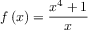in the same manner as in Exercise 58. Then use your results to help sketch the graph of f.

23 May
Mathematics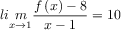22 May
Mathematics

Find the functions (a)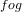(b)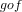, (c)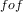, and (d)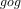.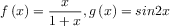21 May
Mathematics

Use part 1 of the Fundamental Theorem of Calculus to find the derivative of the function.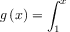24H
Mathematics

Evaluate the integral, if it exists.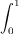22 May
Mathematics

Suppose that the only information we have about a function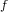is that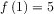and the graph of its derivative is as shown

(a) Use a linear approximation to estimate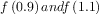.

(b) Are your estimates in part (a) too large or too small? Explain.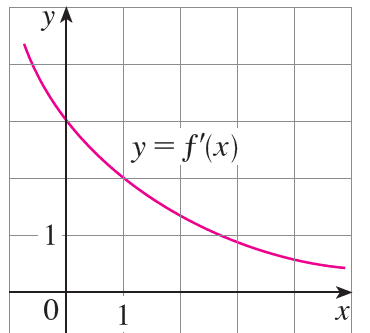24H
Mathematics

Evaluate the integral.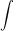26 May
Mathematics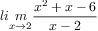24 May
Mathematics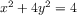25 May
Mathematics

Use Definition 2 to find an expression for the area under the graph of f as a limit. Do not evaluate the limit.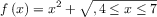24H
Mathematics

Find the first and second derivatives of the function.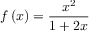24 May
Mathematics

Graph the function by hand, not by plotting points, but by starting with the graph of one of the standard functions given in Section 1.2, and then applying the appropriate transformations.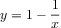13 May 2020
###### Mathematics Homework Help

When you have a math problem that you can't solve, 24/7 Mathematics Homework Help from OneClass provides step-by-step solutions in less than 24 hours.

###### How to Get Mathematics Homework Answers

It's never been easier to get mathematics homework help. Students can simply snap a photo of the math problem that they're struggling with. From this uploaded photo, online math tutors will prepare a detailed solution to your homework problem. If a photo isn't convenient, you can also get help with your mathematics homework by typing your question or by using the online formula builder.

###### Mathematics Experts Are Available 24/7

With 24/7 Mathematics Homework Help, all solutions are written by online tutors who have graduate-level mathematics experience or higher. Tutors are available around the clock, and you can always check online to see how many tutors are actively assisting students.

Mathematics may be one of the most difficult subjects in school. In fact, only 33 percent of eighth-graders are proficient in math at their grade level1, and only 50 percent have a positive view of the subject2. With on-demand access to math homework answers, you're never far from the knowledge you need to get the grades you want.

###### What Type of Mathematic Homework Is Supported?

Both high school and college students can use OneClass to get mathematics homework answers. There's no limit to the type of question you can ask, and tutors can help with everything from mathematical word problems to formula calculations. Students commonly ask about algebra, geometry, trigonometry, pre-calculus, calculus, and more.